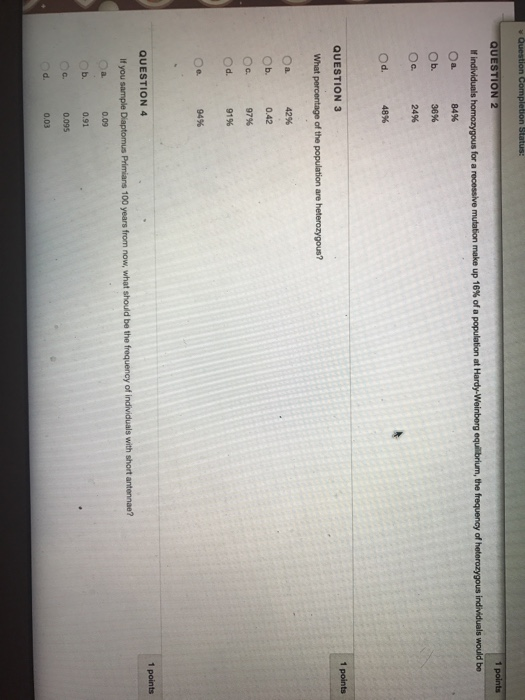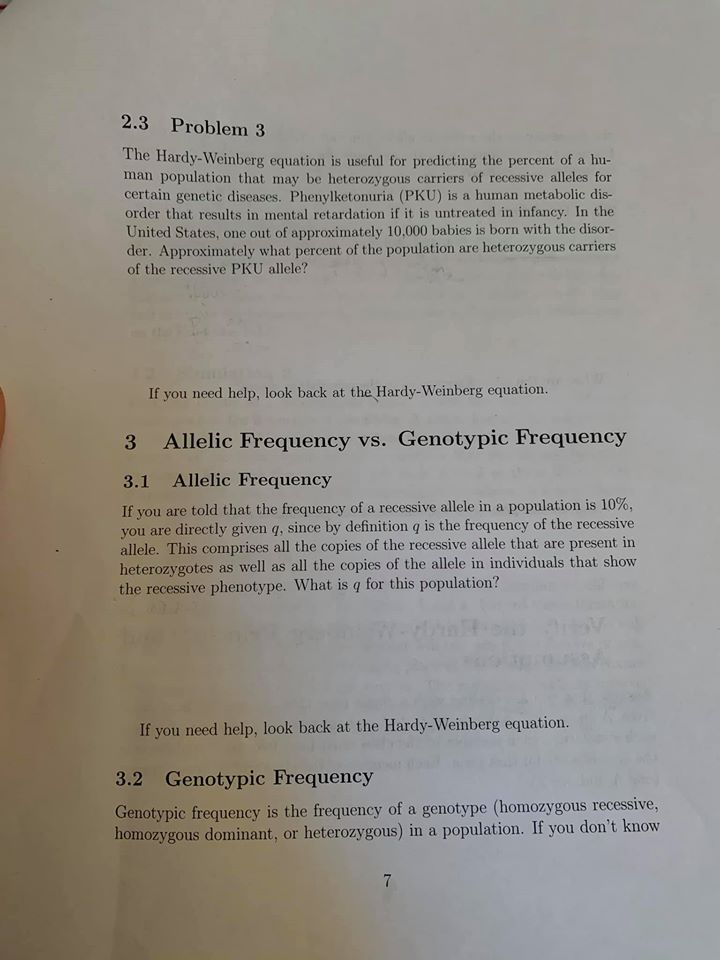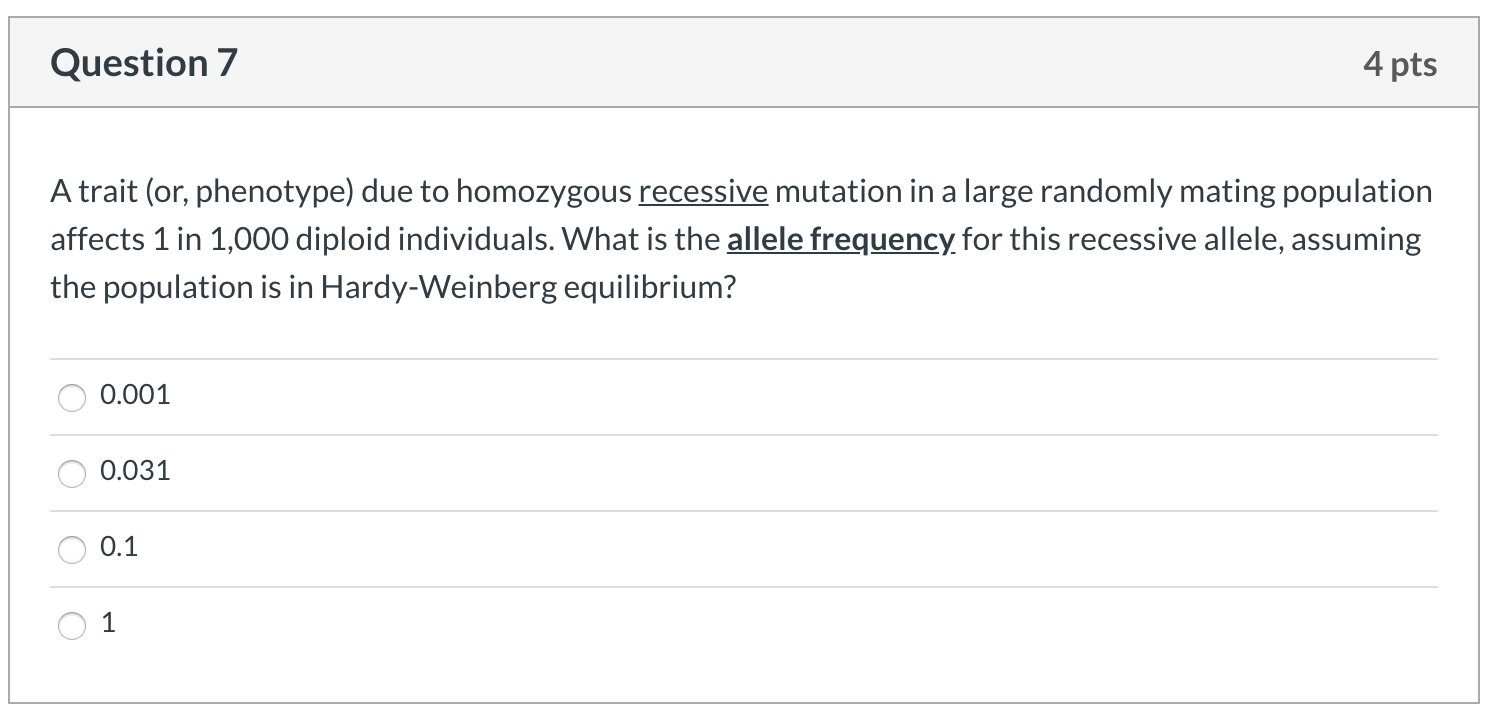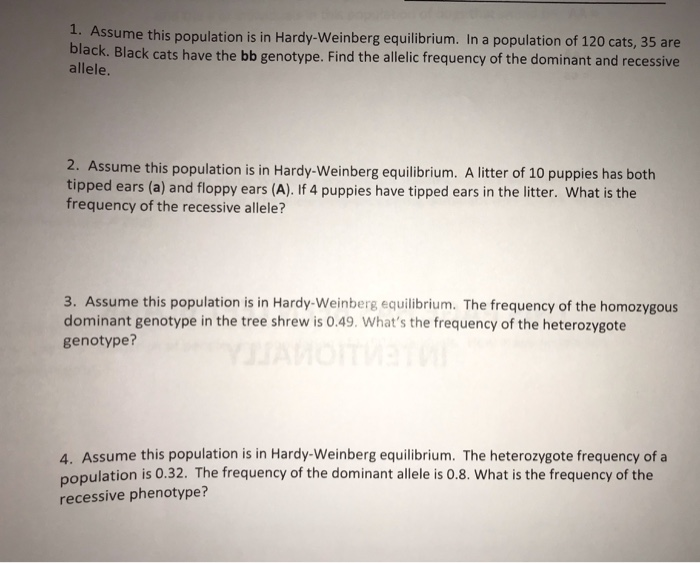QuestionIndividual homozygous dominant for a population at 16 percent recessive would make up

p2 + 2pq + q2 = 1

p + q = 1

0.16 + q = 1

q = 1- 0.16

= 0.84 i.e 84 % (dominant)

1. Hence the frequency of heterozygous population will be:

2 p x q

2 x 0.16 x 0.84 = 0.2688 i.e ( c ) 24% approx

1. Percentage of heterozygous will be:
2. 24%

3. Diamptomous priminas frequency with short antenna:

(0.09) (a)

#### Earn Coins

Coins can be redeemed for fabulous gifts.

Similar Homework Help Questions
• ### In a population that is in Hardy Weinberg equilibrium, 49% of the individuals are recessive homozygotes...

In a population that is in Hardy Weinberg equilibrium, 49% of the individuals are recessive homozygotes for a certain trait. In a population of 5500, calculate the following: a) The number of homozygous recessive individuals in the population b) The number of heterozygotes in the population

• ### Consider a randomly-mating population that is not evolving. If the population consists of 16% homozygous recessive...

Consider a randomly-mating population that is not evolving. If the population consists of 16% homozygous recessive individuals, what percentage of the population is heterozygous? A. 84% B. 50% C. 42% D. 48%

• ### Use the Hardy-Weinberg equations (p2 + 2pq + q2 = 1 and p + q =...

Use the Hardy-Weinberg equations (p2 + 2pq + q2 = 1 and p + q = 1) to find the genotypes of the following hypothetical phenotypic information: a.       Within a particular population, you observe that 450 individuals have freckles (dominant, F) and 550 do not have freckles (recessive, f). b.      Homozygous dominant (frequency) ______(A)_________ c.       Heterozygous (frequency) ______(B)_____________ d.      Homozygous recessive (frequency) _______(C)____________

• ### 2.3 Problem 3 The Hardy-Weinberg equation is useful for predicting the percent of a hu- man...2.3 Problem 3 The Hardy-Weinberg equation is useful for predicting the percent of a hu- man population that may be heterozygous carriers of recessive alleles for certain genetic diseases. Phenylketonuria (PKU) is a human metabolic dis- order that results in mental retardation if it is untreated in infancy. In the United States, one out of approximately 10.000 babies is born with the disor- der. Approximately what percent of the population are heterozygous carriers of the recessive PKU allele? If you...

• ### 2. Hardy-Weinberg Equilibrium; chi-square test Sickle cell anemia is a recessive disorder caused by a recessive...

2. Hardy-Weinberg Equilibrium; chi-square test Sickle cell anemia is a recessive disorder caused by a recessive mutation (S) in the b-hemoglobin gene. 80% of affected SS individuals die before reproducing.   Heterozygotes (AS) and homozygous dominant (AA) individuals do not have sickle cell anemia. The table below shows the number of people of each genotype in a population of 100 people in population of Cameroon. Observed # individuals in a Cameroon population AA AS SS 62 32 6 What are the...

• ### Question 7 4 pts A trait (or, phenotype) due to homozygous recessive mutation in a large...Question 7 4 pts A trait (or, phenotype) due to homozygous recessive mutation in a large randomly mating population affects 1 in 1,000 diploid individuals. What is the allele frequency for this recessive allele, assuming the population is in Hardy-Weinberg equilibrium? O 0.001 O 0.031 O 0.1 0 1

• ### Equations: p + q=1 p2 + 2(p)(q) + q2 1) In a population with Hardy-Weinberg equilibrium,...

Equations: p + q=1 p2 + 2(p)(q) + q2 1) In a population with Hardy-Weinberg equilibrium, the frequency of a dominant allele for a gene is 85% (0.85) while the frequency of the recessive allele for the same gene is 15% (0.15). 0.0005 0.013 0.675 0.723 0.255 0.50 0.023 2) proportion of individuals will be homozygous recessive? 0.0005 0.013 0.675 0.723 0.255 0.50 0.023 3) proportion of individuals will be homozygous dominant? 0.0005 0.723 0.013 0.675 0.255 0.50 0.023 4)...

• ### 2. Assume a population is in Hardy-Weinberg equilibrium for a given genetic autosomal trait. What proportion...

2. Assume a population is in Hardy-Weinberg equilibrium for a given genetic autosomal trait. What proportion of individuals in the population are heterozygous for the gene if the frequency of the recessive allele is 1%.

• ### Evolution 1. Assume this population is in Hardy-Weinberg equilibrium. In a population of 120 cats, 35...Evolution 1. Assume this population is in Hardy-Weinberg equilibrium. In a population of 120 cats, 35 are black. Black cats have the bb genotype. Find the allelic frequency of the dominant and recessive allele. 2. Assume this population is in Hardy-Weinberg equilibrium. A litter of 10 puppies has both tipped ears (a) and floppy ears (A). If 4 puppies have tipped ears in the litter. What is the frequency of the recessive allele? 3. Assume this population is in Hardy-Weinberg...

• ### Cystic fibrosis is an autosomal recessive disorder. In one population, the frequency of affected individuals (A...Cystic fibrosis is an autosomal recessive disorder. In one population, the frequency of affected individuals (A 2 A 2) is 0.0004 Assuming that this population is under Hardy- Weinberg equilibrium, calculate all allele frequencies and genotype frequencies. Enter your numerical answers in the boxes below. Express each answer to four decimal places. Frequency of A1: Frequency of A2: Frequency of homozygous dominant: Frequency of homozygous recessive: Frequency of heterozygous